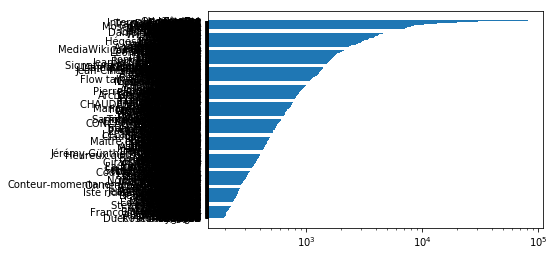What is the annual volume of patrolling? Does user activity follow a power law?

Read log entries from a replica database.

import pymysql  # We will use pymysql to connect to the database
import os

host = os.environ['MYSQL_HOST']

conn = pymysql.connect(
host=host,
user=user,
)

with conn.cursor(pymysql.cursors.DictCursor) as cur:
cur.execute('use frwiki_p')
cur.execute("""
SELECT *
FROM logging
WHERE log_timestamp BETWEEN '20170620000000' AND '20180620000000'
-- WHERE log_timestamp BETWEEN '20180618000000' AND '20180620000000'
""")

result = cur.fetchall()

# TODO: store to a file; try read before querying.


Extract a list of authors for the log entries.

authors = [row["log_user_text"].decode("utf-8", "replace") for row in result if row["log_user_text"] is not None]


Graph the number of patrolling logs for each contributor during the time window, on a log scale so that the power law is evident.

%matplotlib inline
from collections import Counter
from matplotlib import pyplot as plt
import numpy as np

counts = Counter(authors).most_common(1000)

print(np.array(counts))

# So that pyplot is descending order.
counts.reverse()

pos = np.arange(len(counts))
plt.barh(
pos,
[c for c in counts],
tick_label=[c for c in counts],
log=True
)
plt.show()

[['HarrietaCat' '81355']
['OrlodrimBot' '63191']
['Lomita' '48980']
...
['Ludo29' '196']
['Duet Paris By Night' '195']
['Alchemica' '193']]